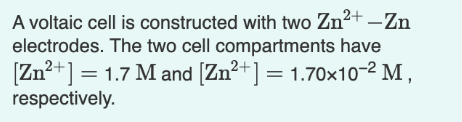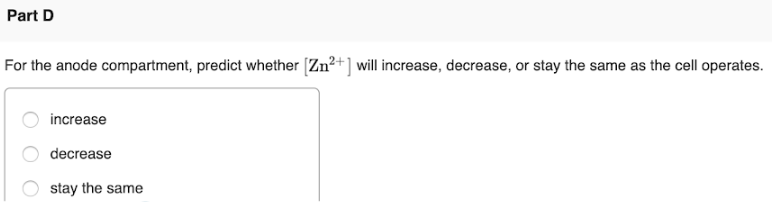# A voltaic cell is constructed with two Zn^2+ -Zn electrodes. The two cell compartments have [Zn^2+] = 1.7 M and [Zn^2+] = 1.70x10^-2M, respectively. For the anode compartment, predict whether [Zn^2+] will increase, decrease, or stay the same as the cell operates. a) increase b) decrease c) stay the same